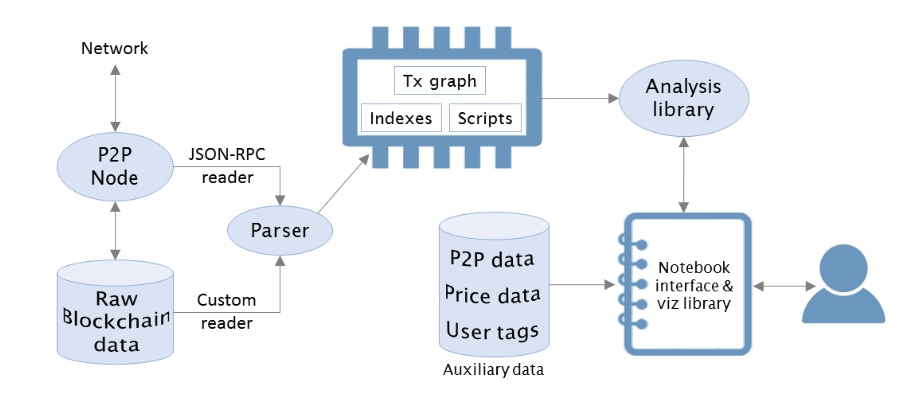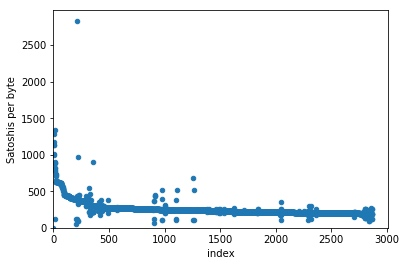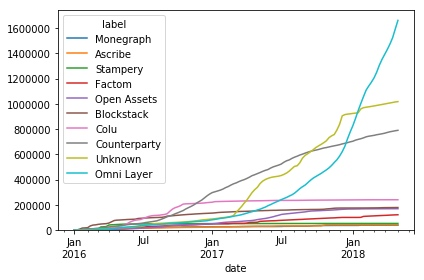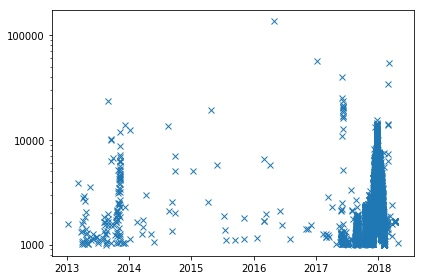## 安装BlockSci框架

BlockSci的主要开发语言是C++，本地编译需要GCC 7.2+和CLang 5+。 官方文档仅提供了Ubuntu 16.04和Mac OS10.13下的本地安装方法， 其他系统可以尝试本地安装或使用官方提供的AWS EC2镜像

## BlockSci框架概述

BlockSci包含两个主要的组件：解析器和分析库。

• 解析器用来解析区块链数据并转换为便于执行分析任务的BlockSci数据格式。
• 分析库则用来编写数据分析任务。## BlockSci解析器 / Parser

BlockSci的解析器支持两种处理原始区块链数据的机制：磁盘模式和RPC模式。

• 磁盘模式是处理比特币区块数据的推荐模式，它可以直接读取并解析磁盘上的比特币区块数据文件，因此速度要快得多。但是这也意味着磁盘模式不能支持更多的区块链，因为不同的区块链的磁盘数据文件格式不同。

使用以下命令以磁盘模式解析比特币区块数据：

``blocksci_parser --output-directory bitcoin-data update disk --coin-directory .bitcoin``

参数说明：

• –output-directory：解析结果输出目录
• –coin-directory：bitcoin core数据目录
• RPC模式使用数据加密货币区块链节点的RPC接口来提取数据，而RPC接口是大多数（山寨币）区块链都支持的与比特币类似的二次开发接口（虽然优势也有一些差异），因此在比特币之外的区块链数据解析，BlockSci通常采用RPC模式，例如对于Zcash和Namecoin。

要使用RPC模式的BlockSci解析器，需要一个区块链全节点并且启用txindex选项。 命令格式如下：

``blocksci_parser --output-directory bitcoin-data update rpc --username [user] --password [pass] --address [ip] --port [port]``

参数说明：

• –output-directory：解析结果输出目录
• –port：RPC API监听端口

## BlockSci分析库 / Analysis Library

``````#include &lt;blocksci/blocksci.hpp>

int main(int argc, const char * argv[]) {
blocksci::Blockchain chain{"file_path_to_output-directory"};
}``````

BlockSci目前仅支持Python 3，使用方法很简单：导入BlockSci库，然后利用解析器输出目录的数据构建Blockchain对象：

``````import blocksci
chain = blocksci.Blockchain("file_path_to_parser_output-directory")``````

## BlockSci区块链数据分析示例

``````import blocksci
import matplotlib.pyplot as plt
import matplotlib.ticker
import collections
import pandas as pd
import numpy as np

chain = blocksci.Blockchain('your_parser_data_directory')``````

### 比特币地址类型使用情况分类统计

``````net_coins_per_block = chain.map_blocks(lambda block: block.net_address_type_value())

df = pd.DataFrame(net_coins_per_block).fillna(0).cumsum()/1e8
df = chain.heights_to_dates(df)
df = df.rename(columns={t:str(t) for t in df.columns})
ax = df.resample("W").mean().plot()
ax.set_ylim(ymin=0)``````

### 比特币单区块交易手续费率可视化分析

``````example_block_height = 465100
df = pd.DataFrame(chain[example_block_height].txes.fee_per_byte(), columns=["Satoshis per byte"])
ax = df.reset_index().plot.scatter(x="index", y="Satoshis per byte")
ax.set_ylim(0)
ax.set_xlim(0)
plt.show()``````### 比特币二层协议使用情况可视化分析

``````txes = chain.blocks.txes.including_output_of_type(blocksci.address_type.nulldata).all
labels = [(tx.block.time, blocksci.label_application(tx)) for tx in txes]

df = pd.DataFrame(labels, columns=["date", "label"])
df = df.reset_index().groupby(["date", "label"]).count().unstack(level=-1).fillna(0)
df.columns = df.columns.droplevel()
important_columns = list(df[df.index > pd.to_datetime("1-1-2016")].sum().sort_values()[-10:].index)
important_columns = [x for x in important_columns if "Address" not in x]
ax = df[df.index > pd.to_datetime("1-1-2016")].cumsum().resample("w").mean()[important_columns].plot()
ax.set_ylim(0)
plt.tight_layout()``````### 比特币2017年各月平均交易手续费可视化分析

``````converter = blocksci.CurrencyConverter()
blocks = chain.range('2017')
times = blocks.time

df = pd.DataFrame({"Fee":fees}, index=times)
df = converter.satoshi_to_currency_df(df, chain)
ax = df.resample("d").mean().plot(legend=False)
ax.set_ylim(ymin=0)
plt.tight_layout()``````

### 比特币大额手续费交易可视化分析

``````high_fee_txes = chain.cpp.filter_tx("fee(tx) > 10000000", 0, len(chain))
converter = blocksci.CurrencyConverter()

df = pd.DataFrame([(tx.block.height, tx.fee) for tx in high_fee_txes], columns=["height", "fee"])
df.index = df["height"]
df["fee"] = df.apply(lambda x: converter.satoshi_to_currency(x["fee"], chain[x["height"]].time), axis=1)
df = df[df["fee"] > 1000]
df = chain.heights_to_dates(df)
fig, ax = plt.subplots()
ax.plot_date(df.index, df["fee"], fmt="x")
ax.set_yscale("log")
formatter = matplotlib.ticker.ScalarFormatter(useOffset=False)
formatter.set_scientific(False)
ax.yaxis.set_major_formatter(formatter)
plt.tight_layout()``````2017年超过1000美元手续费的交易激增，从一个侧面说明了这一年涌入了大量数字加密货币的新手。

• 发表于 2020-01-07 15:01
• 阅读 ( 1353 )
• 学分 ( 15 )
• 分类：其他